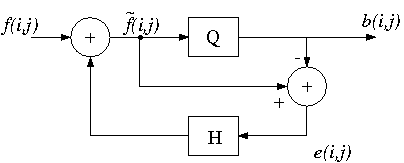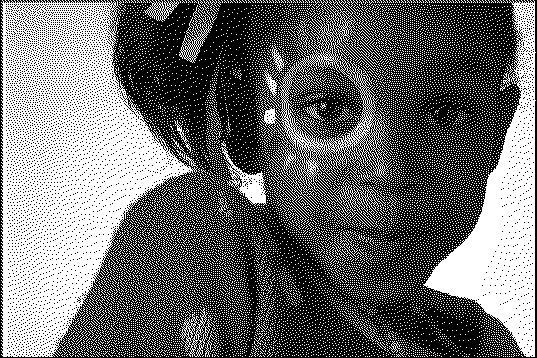# 0.16 Lab 10b - image processing (part 2)  (Page 5/5)

 Page 5 / 5Block diagram of the error diffusion method.

[link] is a block diagram that illustrates the method of error diffusion.The current input pixel $f\left(i,j\right)$ is modified by means of past quantization errors to give a modified input $\stackrel{˜}{f}\left(i,j\right)$ . This pixel is then quantized to a binary value by $Q$ , using some threshold $T$ . The error $e\left(i,j\right)$ is defined as

$e\left(i,j\right)=\stackrel{˜}{f}\left(i,j\right)-b\left(i,j\right)$

where $b\left(i,j\right)$ is the quantized binary image.

The error $e\left(i,j\right)$ of quantizing the current pixel is is diffused to "future" pixels by means of a two-dimensionalweighting filter $h\left(i,j\right)$ , known as the diffusion filter . The process of modifying an input pixel by past errors can berepresented by the following recursive relationship.

$\stackrel{˜}{f}\left(i,j\right)=f\left(i,j\right)+\sum _{k,l\in S}h\left(k,l\right)e\left(i-k,j-l\right)$

The most popular error diffusion method, proposed by Floyd and Steinberg, uses the diffusion filter shown in [link] . Since the filter coefficients sum to one,the local average value of the quantized image is equal to the local average gray scale value. [link] shows the halftone image produced by Floydand Steinberg error diffusion. Compared to the ordered dither halftoning, the error diffusion method can be seen to have better contrast performance.However, it can be seen in [link] that error diffusion tends to create "streaking" artifacts, known as worm patterns.The error diffusion filter proposed by Floyd and Steinberg.A halftone image produced by the Floyd and Steinberg error diffusion method.

## Halftoning exercise

Download the file house.tif for the following section.

If your display software (e.g Matlab) resizes your image before rendering, a halftoned image will probably notbe rendered properly. For example, a subsampling filter will result in gray pixels in the displayedimage! To prevent this in Matlab, use the truesize command just after the image command. This will assign one monitor pixel for each image pixel.

We will now implement the halftoning techniques described above. Savethe result of each method in MAT files so that you may later analyze and compare their performance.Download the image file house.tif and read it into Matlab. Print out a copy of this image.

First try the simple thresholding technique based on [link] , using $T=108$ , and display the result. In Matlab, an easy way to threshold an image $X$ is to use the command Y = 255*(X>T); . Label the quantized image, and print it out.

Now create an "absolute error" image by subtracting the binary from the original image, and then taking the absolute value.The degree to which the original image is present in the error image is a measure of signal dependence of the quantization error.Label and print out the error image.

Compute the mean square error (MSE), which is defined by

$MSE=\frac{1}{NM}\sum _{i,j}{\left\{f\left(i,j\right)-b\left(i,j\right)\right\}}^{2}$

where $NM$ is the total number of pixels in each image. Note the MSE on the printout of the quantized image.

Now try implementing Bayer dithering of size 4. You will first have to compute the dither pattern. The index matrix fora dither pattern of size 4 is given by

$I\left(i,j\right)=\left[\begin{array}{cccc}12& 8& 10& 6\\ 4& 16& 2& 14\\ 9& 5& 11& 7\\ 1& 13& 3& 15\end{array},\phantom{\rule{4pt}{0ex}},.\right]$

Based on this index matrix and [link] , create the corresponding threshold matrix.

For ordered dithering, it is easiest to perform the thresholding of the image all at once.This can be done by creating a large threshold matrix by repeating the $4×4$ dither pattern. For example, the command T = [T T; T T]; will increase the dimensions of $T$ by 2. If this is repeated until $T$ is at least as large as the original image, $T$ can then be trimmed so that is is the same size as the image. The thresholding can then be performed using the command Y = 255*(X>T); .

As above, compute an error image and calculate the MSE. Print out the quantized image, the error image, and note the MSE.

Now try halftoning via the error diffusion technique, using a threshold $T=108$ and the diffusion filter in [link] . It is most straightforward to implement this by performing the followingsteps on each pixel in raster order:

1. Initialize an output image matrix with zeros.
2. Quantize the current pixel using using the threshold $T$ , and place the result in the output matrix.
3. Compute the quantization error by subtracting the binary pixel from the gray scale pixel.
4. Add scaled versions of this error to “future” pixels of the original image, as depictedby the diffusion filter of [link] .
5. Move on to the next pixel.

You do not have to quantize the outer border of the image.

As above, compute an error image and calculate the MSE. Print out the quantized image, the error image, and note the MSE.

The human visual system naturally lowpass filters halftone images. To analyze this phenomenon, filter each of the halftone images withthe Gaussian lowpass filter $h$ that you loaded in the previous section (from ycbcr.mat ), and measure the MSE of the filtered versions. Make a table that containsthe MSE's for both filtered and nonfiltered halftone images for each of the three methods. Does lowpass filtering reduce the MSE for each method?

## Inlab report

1. Hand in the original image and the three binary images. Make sure that they are all labeled, and that the mean square errors are notedon the binary images.
2. Compare the performance of the three methods based on the visual quality of the halftoned images.Also compare the resultant MSE's. Is the MSE consistent with the visual quality?
3. Submit the three error images. Which method appears to be the least signal dependent? Does the signal dependence seem to becorrelated with the visual quality?
4. Compare the MSE's of the filtered versions with the nonfiltered versions for each method.What is the implication of these observations with respect to how we perceive halftone images.

#### Questions & Answers

Is there any normative that regulates the use of silver nanoparticles?
what king of growth are you checking .?
Renato
What fields keep nano created devices from performing or assimulating ? Magnetic fields ? Are do they assimilate ?
why we need to study biomolecules, molecular biology in nanotechnology?
?
Kyle
yes I'm doing my masters in nanotechnology, we are being studying all these domains as well..
why?
what school?
Kyle
biomolecules are e building blocks of every organics and inorganic materials.
Joe
anyone know any internet site where one can find nanotechnology papers?
research.net
kanaga
sciencedirect big data base
Ernesto
Introduction about quantum dots in nanotechnology
what does nano mean?
nano basically means 10^(-9). nanometer is a unit to measure length.
Bharti
do you think it's worthwhile in the long term to study the effects and possibilities of nanotechnology on viral treatment?
absolutely yes
Daniel
how to know photocatalytic properties of tio2 nanoparticles...what to do now
it is a goid question and i want to know the answer as well
Maciej
characteristics of micro business
Abigail
for teaching engĺish at school how nano technology help us
Anassong
Do somebody tell me a best nano engineering book for beginners?
there is no specific books for beginners but there is book called principle of nanotechnology
NANO
what is fullerene does it is used to make bukky balls
are you nano engineer ?
s.
fullerene is a bucky ball aka Carbon 60 molecule. It was name by the architect Fuller. He design the geodesic dome. it resembles a soccer ball.
Tarell
what is the actual application of fullerenes nowadays?
Damian
That is a great question Damian. best way to answer that question is to Google it. there are hundreds of applications for buck minister fullerenes, from medical to aerospace. you can also find plenty of research papers that will give you great detail on the potential applications of fullerenes.
Tarell
what is the Synthesis, properties,and applications of carbon nano chemistry
Mostly, they use nano carbon for electronics and for materials to be strengthened.
Virgil
is Bucky paper clear?
CYNTHIA
carbon nanotubes has various application in fuel cells membrane, current research on cancer drug,and in electronics MEMS and NEMS etc
NANO
so some one know about replacing silicon atom with phosphorous in semiconductors device?
Yeah, it is a pain to say the least. You basically have to heat the substarte up to around 1000 degrees celcius then pass phosphene gas over top of it, which is explosive and toxic by the way, under very low pressure.
Harper
Do you know which machine is used to that process?
s.
how to fabricate graphene ink ?
for screen printed electrodes ?
SUYASH
What is lattice structure?
of graphene you mean?
Ebrahim
or in general
Ebrahim
in general
s.
Graphene has a hexagonal structure
tahir
On having this app for quite a bit time, Haven't realised there's a chat room in it.
Cied
what is biological synthesis of nanoparticles
how did you get the value of 2000N.What calculations are needed to arrive at it
Privacy Information Security Software Version 1.1a
Good
Got questions? Join the online conversation and get instant answers!By Edward BitonBy Sam LuongBy